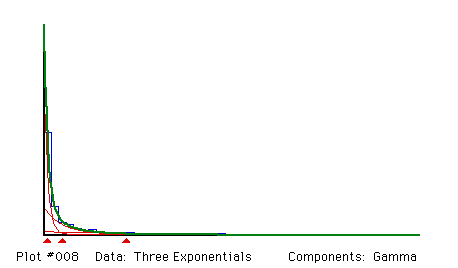## A mixture of three exponential distributions

#### Source:

A total of 1000 observations was generated by computer to follow the mixture distribution
1/3 E(1) + 1/3 E(4) + 1/3 E(16)
where E(m ) denotes an exponential distribution with mean m .

#### Remarks:

Exponential distributions are fitted by constraining gamma distributions to have unit coefficient of variation. Mixtures of exponential distributions are always unimodal and skewed, and components with large mean completely overlap the components with small mean. As a result, fitting a mixture of exponential distributions is often a frustrating experience. In many applications, it may be impossible to estimate all of the proportions and all of the means; in this example it was possible, but all of the estimates have rather large standard errors, despite the large sample size. MIX does not plot exponential mixtures very elegantly.``` Fitting Gamma components

Proportions and their standard errors
.47942    .36121    .15937
.08481    .06844    .05163

Means and their standard errors
.9770    4.8888   22.0166
.2148    1.4076    4.5749

Sigmas (FIXED COEF. OF VAR. =  1.0000)
.9770    4.8888   22.0166

Degrees of freedom = 25 - 1 +   0 -  0 -  5 -   0 =  19

Chi-squared =  21.4570            (P =  .3121)```

Back to the MIX Demonstration Examples Page